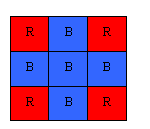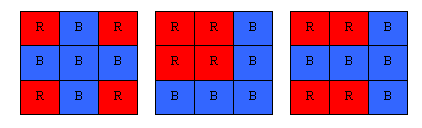#### You may also like### Number Detective

Follow the clues to find the mystery number.### Make 37

Four bags contain a large number of 1s, 3s, 5s and 7s. Pick any ten numbers from the bags above so that their total is 37.### Prime Magic

Place the numbers 1, 2, 3,..., 9 one on each square of a 3 by 3 grid so that all the rows and columns add up to a prime number. How many different solutions can you find?

# Red Even

##### Age 7 to 11 Challenge Level:

Correct solutions were received from Holly (Nottingham High School for Girls), Andrei (School 205 Bucharest) and Michael (home educated).

I liked Holly's explanation. It is hard to write the solution to such a visual problem and it showed a lot of insight so I have included it here.

The answer to the first part is 1, because there are, logically, only three places that the first red counter could go:

• the middle,
• the middle of an edge or
• a corner.

If a red counter was put in the middle then two more would be needed to go in corners (to give the diagonals an even number), and two to go on the middle column and the middle row. This is five red counters which are not available.
If a counter was placed in the middle of an edge then one would be needed on a corner, and then another to go on a diagonal and another to go along the side. The one along the side would then need another in its column/row, again 5 red counters.
However, if one were placed in a corner then another would be placed in that row and another in that column. the only way to do it, to suit the diagonals, is to have a red counter in each corner.If the diagonals are not included, then two additional ways are possible. To create a solution the board must have symmetry, this meaning that the only other ways possible are having all four in one corner or having two on each side(opposite each other). If there was not symmetry then the counters would not work because each counter needs two more on its row and column, therefore they must also be able to form a square or a rectangle.